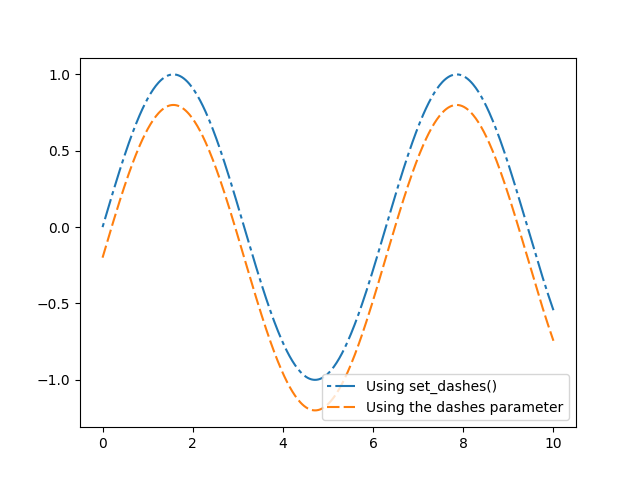# Customizing dashed line styles¶

The dashing of a line is controlled via a dash sequence. It can be modified using `Line2D.set_dashes`.

The dash sequence is a series of on/off lengths in points, e.g. `[3, 1]` would be 3pt long lines separated by 1pt spaces.

Some functions like `Axes.plot` support passing Line properties as keyword arguments. In such a case, you can already set the dashing when creating the line.

Note: The dash style can also be configured via a property_cycle by passing a list of dash sequences using the keyword dashes to the cycler. This is not shown within this example.```import numpy as np
import matplotlib.pyplot as plt

x = np.linspace(0, 10, 500)
y = np.sin(x)

fig, ax = plt.subplots()

# Using set_dashes() to modify dashing of an existing line
line1, = ax.plot(x, y, label='Using set_dashes()')
line1.set_dashes([2, 2, 10, 2])  # 2pt line, 2pt break, 10pt line, 2pt break

# Using plot(..., dashes=...) to set the dashing when creating a line
line2, = ax.plot(x, y - 0.2, dashes=[6, 2], label='Using the dashes parameter')

ax.legend()
plt.show()
```

Keywords: matplotlib code example, codex, python plot, pyplot Gallery generated by Sphinx-Gallery